Question

od The capital asset pricing model (CAPM) explains how risk should be considered when stocks and...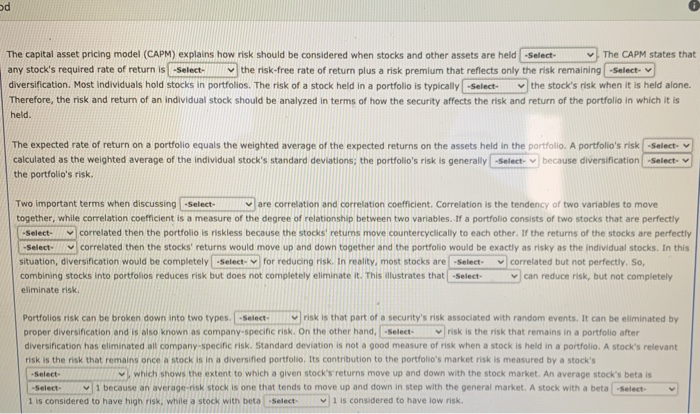od The capital asset pricing model (CAPM) explains how risk should be considered when stocks and other assets are held -Select- The CAPM states that any stock's required rate of return is -Select the risk-free rate of return plus a risk premium that reflects only the risk remaining -Select- diversification. Most individuals hold stocks in portfolios. The risk of a stock held in a portfolio is typically -Select the stock's risk when it is held alone. Therefore, the risk and return of an individual stock should be analyzed in terms of how the security affects the risk and return of the portfolio in which it is held. The expected rate of return on a portfolio equals the weighted average of the expected returns on the assets held in the portfolio. A portfolio's risk -Select- calculated as the weighted average of the Individual stock's standard deviations; the portfolio's risk is generally select because diversification -Select- the portfolio's risk. Two important terms when discussing -Select are correlation and correlation coefficient. Correlation is the tendency of two variables to move together, while correlation coefficient is a measure of the degree of relationship between two variables. If a portfolio consists of two stocks that are perfectly correlated then the portfolio is riskless because the stocks' returns move countereyclically to each other. If the returns of the stocks are perfectly correlated then the stocks' returns would move up and down together and the portfolio would be exactly as risky as the individual stocks. In this situation, diversification would be completely select for reducing risk. In reality, most stocks are -Select correlated but not perfectly. So, combining stocks Into portfolios reduces risk but does not completely eliminate it. This illustrates that -Select can reduce risk, but not completely eliminate risk -Select- -Select- Portfolios risk can be broken down into two types. -Select risk is that part of a security's risk associated with random events. It can be eliminated by proper diversification and is also known as company specific risk. On the other hand, -Select risk is the risk that remains in a portfolio after diversification has eliminated all company-specific risk. Standard deviation is not a good measure of risk when a stock is held in a portfolio. A stock's relevant risk is the risk that remains once a stock is in a diversified portfolio Its contribution to the portfolio's market risk is measured by a stock's Select which shows the extent to which a given stock's returns move up and down with the stock market. An average stock's beta is 1 because an average-risk stock is one that tends to move up and down in step with the general market. A stock with a beta -Select- 1 is considered to have high risk, while a stock with beta -Select 1 is considered to have low risk.

 The CAPM The capital asset pricing model (CAPM) explains how risk should be considered when stocks and other assets are held in portfolios . The CAPM states that any stock's required rate of return isequal to the risk-free rate of return plus a risk premium that reflects only the risk remaining after diversification. Most individuals hold stocks in portfolios. The risk of a stock held in a portfolio is typically lower than the stock's risk when it is held alone. Therefore, the risk and return of an individual stock should be analyzed in terms of how the security affects the risk and return of the portfolio in which it is held. The expected rate of return on a portfolio equals the weighted average of the expected returns on the assets held in the portfolio. A portfolio's risk isn't  calculated as the weighted average of the individual stock's standard deviations; the portfolio's risk is generally smaller because diversification lowers the portfolio's risk Two important terms when discussing portfolio risk are  are correlation and correlation coefficient. Correlation is the tendency of two variables to Two important terms when discussing move together, while correlation coefficient is a measure of the degree of relationship between two variables.  If a portfolio consists of two stocks that are perfectly negatively correalted then the portfolio is riskless because the stocks' returns move countercyclically to each other.  If the returns of  stocks' perfectly positvely  correlated  then the stocks' returns would move up and down together and the portfolio would be exactly as risky as individual stock. In this situation, diversification would be completely useless for reducing risk . In reality, most stocks are positively correlated but not perfectly. So, combining stocks into portfolios reduces risk but does not completely eliminate it.This illustrates that combining stocks into portfolio can reduce risk, but not completely eliminate risk. Portfolios risk can be broken down into two types diversifiable risk is that part of a security's risk associated with random events. It can be eliminated by proper diversification and is also known as company-specific risk. On the other hand, market risk is the risk that remains in a portfolio after diversification has eliminated all company-specific risk. Standard deviation is not a good measure of risk when a stock is held in a portfolio. A stock's relevant risk is the risk that remains once a stock is in a diversified portfolio. Its contribution to the portfolio's market risk is measured by a stock's -Beta cofficient  because an average-risk stock is one that tends to move up and down in step with the stock market. A stock with a beta equal to 1 Higher Lower

Earn Coins

Coins can be redeemed for fabulous gifts.

Similar Homework Help Questions
• 2. 3: Risk and Rates of Return: Risk in Portfolio Context Risk and Rates of Return:...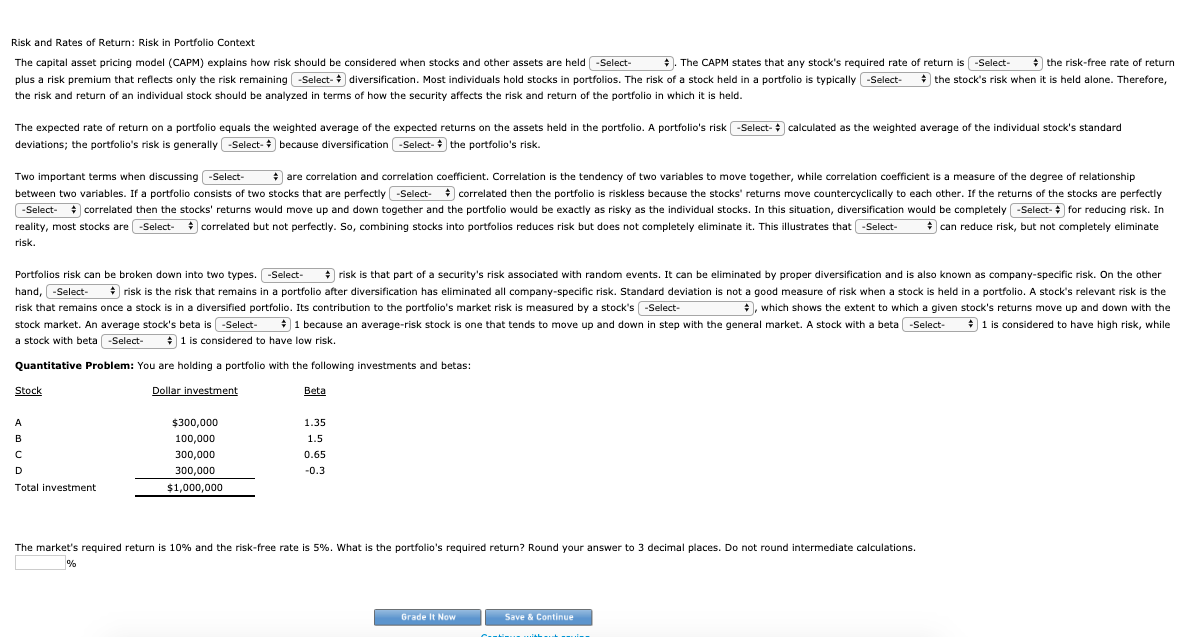2. 3: Risk and Rates of Return: Risk in Portfolio Context Risk and Rates of Return: Risk in Portfolio Context The capital asset pricing model (CAPM) explains how risk should be considered when stocks and other assets are held . The CAPM states that any stock's required rate of return is the risk-free rate of return plus a risk premium that reflects only the risk remaining diversification. Most individuals hold stocks in portfolios. The risk of a stock held in...

• 3. Portfolio risk and diversification Aa Aa E A financial planner is examining the portfolios held...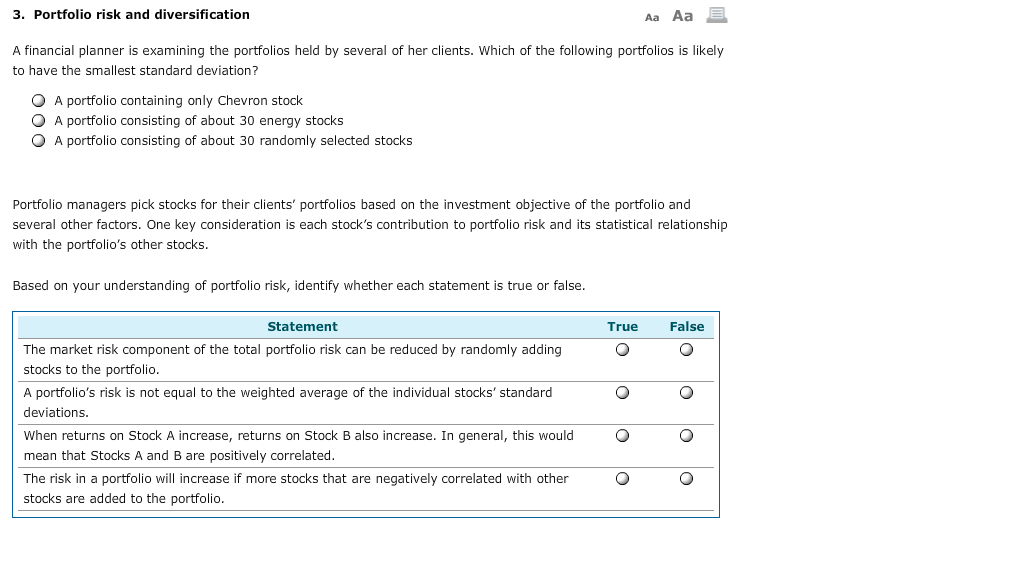3. Portfolio risk and diversification Aa Aa E A financial planner is examining the portfolios held by several of her clients. Which of the following portfolios is likely to have the smallest standard deviation? O O O A portfolio containing only Chevron stock A portfolio consisting of about 30 energy stocks A portfolio consisting of about 30 randomly selected stocks Portfolio managers pick stocks for their clients' portfolios based on the investment objective of the portfolio and several other factors....

• 2. Portfolio risk and diversification A financial planner is examining the portfolios held by several of...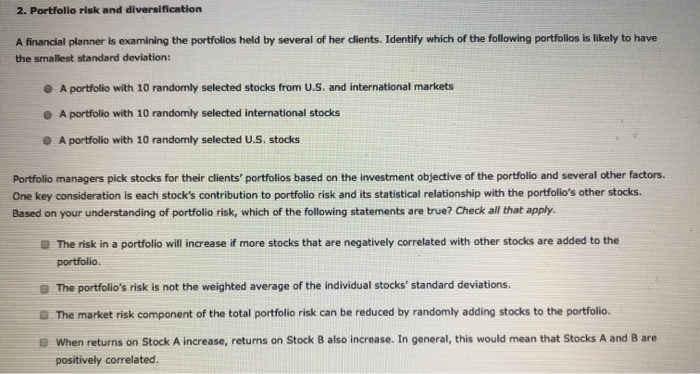2. Portfolio risk and diversification A financial planner is examining the portfolios held by several of her clients. Identify which of the following portfolios is likely to have the smallest standard deviation: A portfolio with 10 randomly selected stocks from U.S. and international markets A portfolio with 10 randomly selected international stocks A portfolio with 10 randomly selected U.S. stocks Portfolio managers pick stocks for their clients' portfolios based on the investment objective of the portfolio and several other factors....

• 3. Portfolio risk and diversification Aa Aa A financial planner is examining the portfolios held by...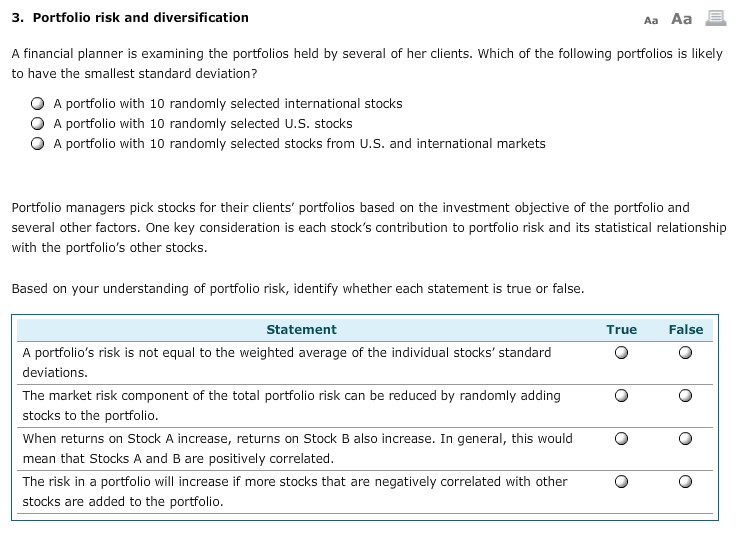3. Portfolio risk and diversification Aa Aa A financial planner is examining the portfolios held by several of her clients. Which of the following portfolios is likely to have the smallest standard deviation ? A portfolio with 10 randomly selected international stocks A portfolio with 10 randomly selected U.S. stocks A portfolio with 10 randomly selected stocks from U.S. and international markets Portfolio managers pick stocks for their clients' portfolios based on the investment objective of the portfolio and several...

• 6. Portfolio risk and diversification A financial planner is examining the portfolios held by several of...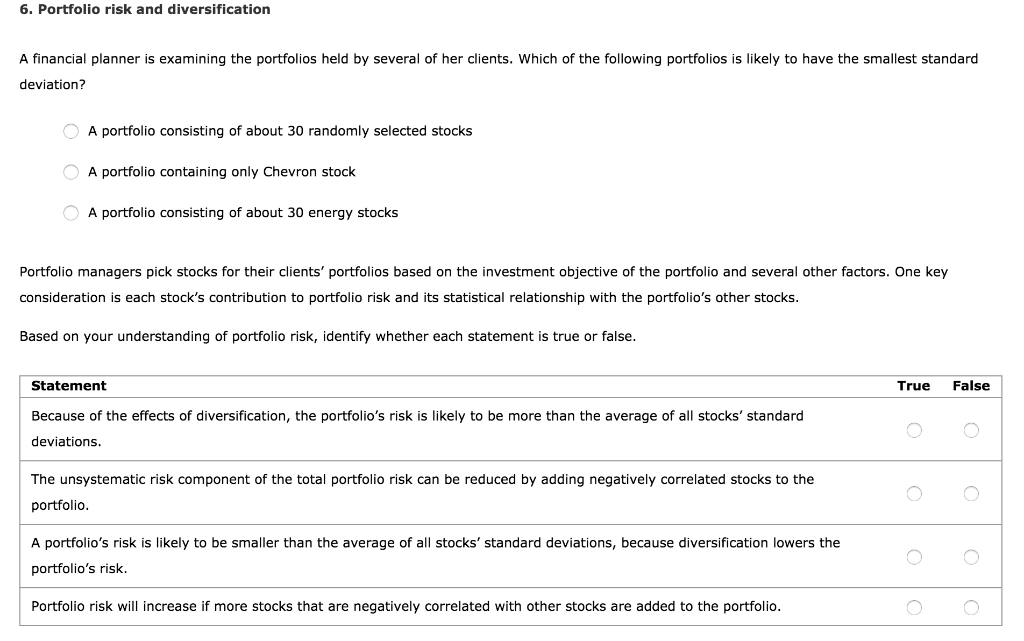6. Portfolio risk and diversification A financial planner is examining the portfolios held by several of her clients. Which of the following portfolios is likely to have the smallest standard deviation? A portfolio consisting of about 30 randomly selected stocks A portfolio containing only Chevron stock A portfolio consisting of about 30 energy stocks Portfolio managers pick stocks for their clients' portfolios based on the investment objective of the portfolio and several other factors. One key consideration is each stock's...

• 3. Portfolio risk and diversification A Aa A financial planner is examining the portfolios held by several of her c...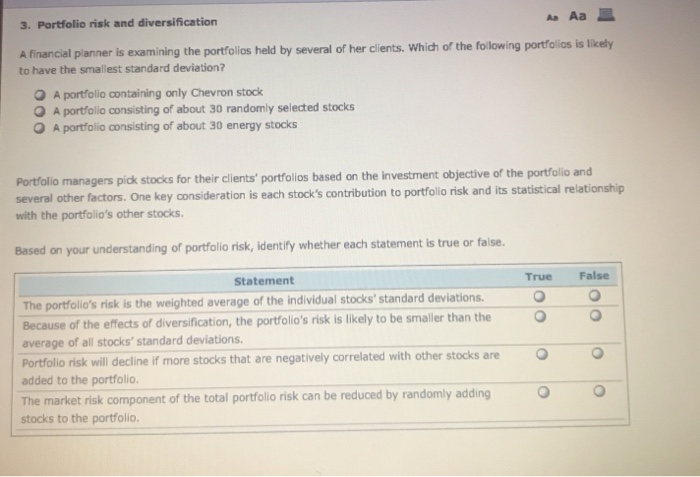3. Portfolio risk and diversification A Aa A financial planner is examining the portfolios held by several of her clients. Which of the following portfolios is likely to have the smallest standard deviation? A portfolio containing only Chevron stock A portfolio consisting of about 30 randomly selected stocks A portfolio consisting of about 30 energy stocks Portfolio managers pick stocks for their clients' portfolios based on the investment objective of the portfolio and several other factors. One key consideration is...

• Assume you wish to evaluate the risk and return behaviors associated with various combinations of two​ stocks, Alpha Sof...

Assume you wish to evaluate the risk and return behaviors associated with various combinations of two​ stocks, Alpha Software and Beta​ Electronics, under three possible degrees of​ correlation: perfect​ positive, uncorrelated, and perfect negative. The average return and standard deviation for each stock appears​ here: Asset   Average Return,overbar r                   Risk (Standard Deviation), s Alpha   5.1%   30.3% Beta                    11.2%      50.5% a. If the returns of assets Alpha and Beta are perfectly positively correlated​ (correlation coefficient equals plus 1​),...

• CAPM, portfolio risk, and return Consider the following information for three stocks, Stocks A, B...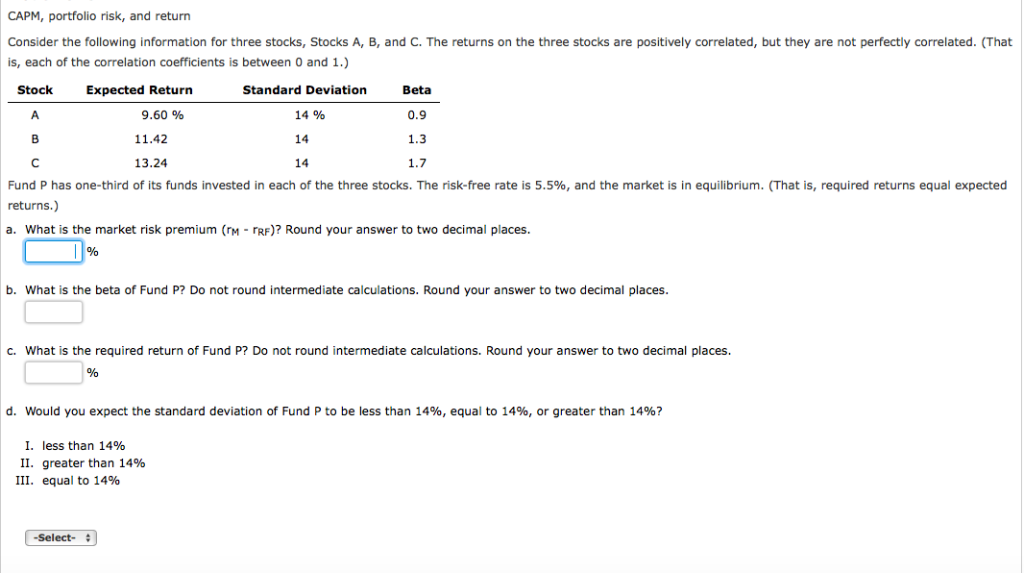CAPM, portfolio risk, and return Consider the following information for three stocks, Stocks A, B, and C. The returns on the three stocks are positively correlated, but they are not perfectly correlated. (That is, each of the correlation coefficients is between 0 and 1.) Stock Standard Deviation 14% 14 14 Beta 0.9 1.3 1.7 Expected Return 9.60 % 11.42 13.24 Fund P has one-third of its funds invested in each of the three stocks. The risk-free rate is 5.5%, and...

• A financial planner is examining the portfolios held by several of her clients. Which of the...

A financial planner is examining the portfolios held by several of her clients. Which of the following portfolios is likely to have the smallest standard deviation? a.) A portfolio's containing Microsoft, Apple, and Google stock b.) A portfolio consisting of about three randomly selected stocks from different sectors. c.) A portfolio containing only Microsoft stock. Portfolio managers pick stocks for their clients' portfolios based on the investment objective of the portfolio and several other factors. One key consideration is each...

• Consider the equation for the Capital Asset Pricing Model (CAPM): îi = rrF + (îm-PRE) *...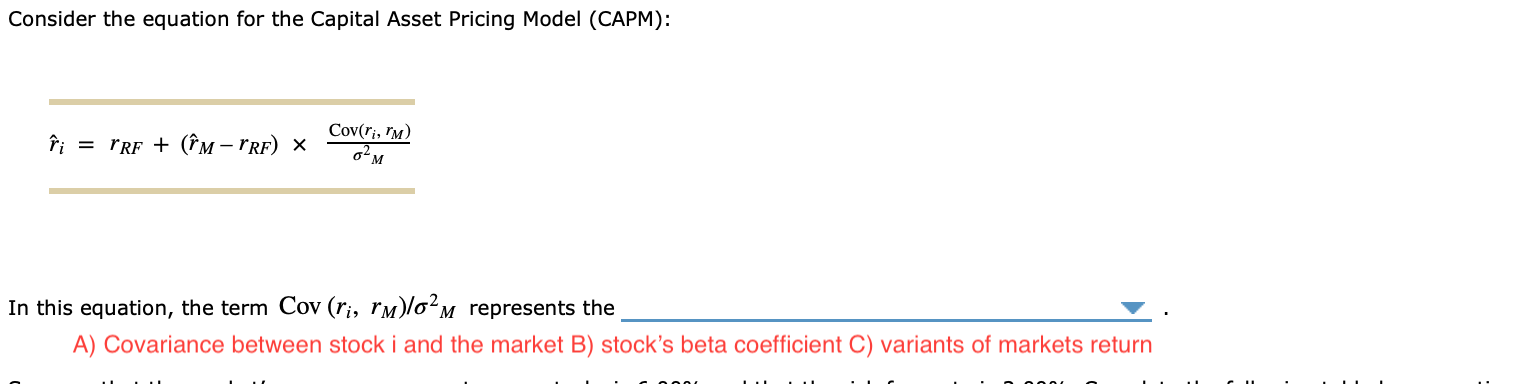Consider the equation for the Capital Asset Pricing Model (CAPM): îi = rrF + (îm-PRE) * Cov(ļi, "M) 02M In this equation, the term Cov (ri, rm)lo?m represents the A) Covariance between stock i and the market B) stock's beta coefficient C) variants of markets return Suppose that the market's average excess return on stocks is 6.00% and that the risk-free rate is 2.00%. Complete the following table by computing expected returns to stocks for each beta coefficient using the...